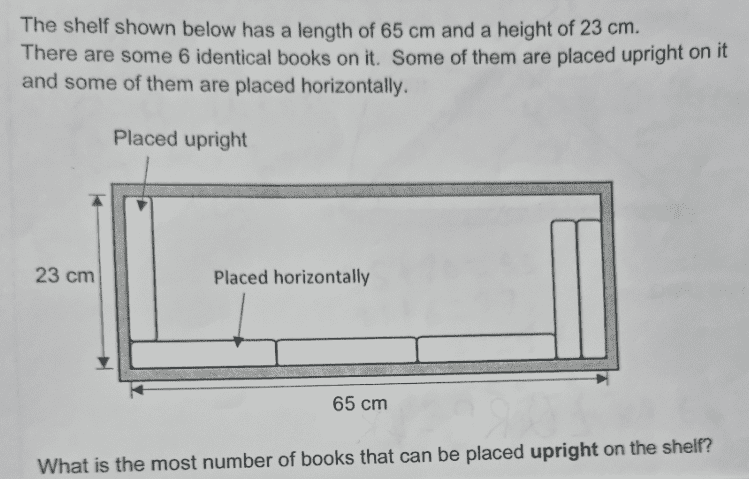# QuestionPls help thanks.

Source: assignment

Let H be the width of the book when placed horizontally.

Let V be the width of book when placed vertically.

3H + 2V = 65
=> (1) H + 2(H + V) =65

Now, we also have (2) H + V = 23

Substituting (2) into (1), we get
H + 2(23) = 65
H = 65 – 46 = 19
So V = 23 – 19 = 4

So the number of books that can be placed upright is 65/V = 65/4 = 16.25 or 16 #

0 Replies 1 Like